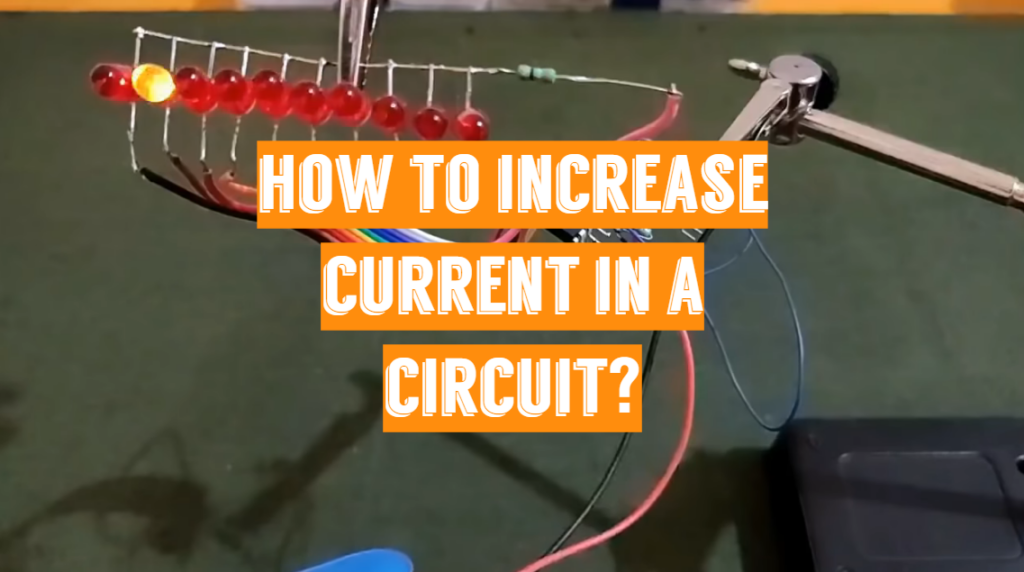# How to Increase Current in a Circuit?

The voltage that drives the current and the resistance that resists it determines the amount of electric current that flows through a circuit. The current flow is understood such that circuits are engineered for a certain voltage and resistance. Heat loss from an excessive current can destroy the circuit, burn the resistors, and even burn the nearby materials.

How to increase current in a circuit, consider two things – amperage and voltage.

A circuit’s electrical current is measured in amperes. The sum of amperes in a circuit is regulated by two factors: volts and resistance. `E/R = A` is the formula for measuring amperage, where E is the voltage applied to a circuit and `R` is the circuit’s resistance.

Related Review: Best Circuit Testers

Take this case. The movement of water into a pipe is similar, with voltage representing the energy pushing the water and resistance representing the pipe’s height. More water runs as more energy is added. The wider the tubing, the lower the resistance and the more water can pass into it.

## 2 ways to increase the current in a circuit

1. Raise the voltage while maintaining the resistance;
2. Lowering the resistance while maintaining a steady voltage;

Boost the supply voltage for a set load. Increase the load with a set supply voltage. To avoid misunderstanding, “increasing a load” refers to lowering the resistance (in the case of a DC circuit) or lowering the impedance (when it comes to an AC power circuit).

The relationship involving voltage, amperage, and resistance in a circuit is described by Ohm’s Law. These 3 properties are inextricably linked; any alteration in one directly affects the other two. `Amperage (I)` multiplied by the sum, or degree, of `resistance (R)` equals `Voltage (V)`. The following theorem, defined as Ohm’s Law, describes how these 3 variables are connected mathematically: `V = IR`. As a result, increasing the amperage of an electric circuit may be accomplished in 2 ways.

Since voltage equals amperage multiplied by resistance in a circuit, the amperage through the circuit may rise if the voltage stays unchanged while the resistance decreases. Increase the size of the conductors in an electrical circuit to minimize resistance, i.e. use bigger diameter copper conductors.

Following the previous example with water pipes, if the electrical circuit includes resistors, resistance may be reduced by switching to a lower-rated resistor, such as a 4-ohm resistor to a 2-ohm resistor. As the resistance in a circuit is reduced in half while the voltage remains constant, the amperage through the circuit is doubled.

Related Reviews:

The amperage in a circuit may be improved by changing the voltage if the circuit’s resistance stays unchanged. If the electrical circuit were a water pipe, the voltage would reflect the water strain, the resistance would represent the pipe’s width, and the amperage would represent the volume of water flowing through the pipe per time period. The volume of water passing into the pipe would double if the pipe was constant and the water pressure doubled.

To maximize amperage, ensure that all of the wiring and electronics will handle a higher current. The sum of amperage that cables, breakers, and other associated equipment can carry is referred to as an “ampere rating”.

If your circuit includes resistor chips, you can reduce resistance and thereby raise amperage by replacing the current resistor with a lower-rated one. You should replace a 6-ohm resistor with a 4-ohm resistor if the current one is 6 ohms. You will raise the circuit’s amperage by maintaining the voltage constant but decreasing the resistance.

How to increase current without altering the voltage:

The significance of current is determined by the circuit’s resistance:

`V = IR`

`R = ρ L/A`

Temperature, cross-sectional area, wire’s length, and other variables all affect resistance. To raise the circuit’s current, you should do the following:

Select a low-resistance conductor, ρ.

1. Make use of a short conductor;
2. Make use of dense wire;
3. Reducing the circuit’s temperature is a good idea;
4. If the operating temperature is high, a semiconductor can be used since it has a negative temperature coefficient;
5. Reduce circuit errors as much as possible;
6. Remove any circuitry that isn’t needed;

## How to increase the output voltage of Arduino Uno?

The case is simple. For instance, you need to increase the output voltage of your Arduino Uno from 5V to 10V. What to do?

An external circuit with its 10V power supply is needed.

You may use the signal to activate a transistor and have 10V to the output of the external circuit when Arduino outputs a logical 1 (5V).

You could also use a diode pump, which is a special configuration of condensers and diodes that multiplies the input voltage by two. You’d like to program the Arduino board to produce a square wave (keep in mind – AC signal with 5V amplitude). The diode pump’s performance would be 10VDC.

The system, on the other hand, is not quite capable of delivering a lot of intensity, so it may or may not have the desired impact depending on the load.

You may also use the Arduino to produce an AC voltage, which you can then feed into a transformer and rectify on the secondary. You might get 10V or about everything else with the right transformer. You won’t be able to produce a million volts signal without additional power sources because transformers aren’t 100 percent effective. I’m sure there’s a cap, but I’m not sure how to figure that out.

Click to rate this post!
[Total: 0 Average: 0]

1.